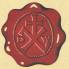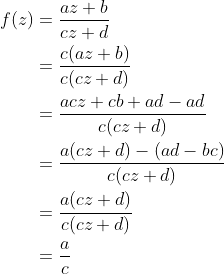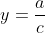Wacker Art Further Functions IBild: "Edersee - Alte Brücke Scheid-Bringhausen"

## Mathematical Functions

### f(x) = sin(12x)cos(x)

Bild: "Alte Baumstümpfe aus dem Edersee"

### f(x) = sin(1/x)

Bild: "Durchbruch Hammerberg"

## Möbius Transformation

The Möbius transformation is defined by the following formular:with complex parameter a,b,c,d and one complex variable z.

If ad-bc = 0 and c≠0; the result of the transformation will be the constant value a/c.This implies the following condition for the parameter a,b,c,d of the Möbius transformation:With this condition we have the Möbius transformation definded.

As next step we have some examples with Möbius transformations with real values. We will have the real valued parameter a,b,c,d and the real valued variable x.Asymtotic behavior for x → ∞ or x → -∞. For these limits the following constant y value will be approached:If the denominator is zero the function has a pole (c ≠ 0); with the following x value:Values of the functions at x = 0.x value for y=0### Examples:

Parameter settings of the examples and the resulting values of the Möbius transformation.

 a b c d Determinant Asymptote y Value Pole x Value y=0 at x y Value at x=0 1 1 1 -1 -2 1 1 -1 -1 1 1 -1 1 2 -1 1 -1 1 1 -1 1 1 2 1 -1 1 -1 -1 1 1 1 -2 -1 -1 1 1

### y = x/(1-x2)

Bild: "Bootssteg"

Next Page:

7. März 2021 Version 2.0
Copyright: Hermann Wacker Uhlandstraße 10 D-85386 Eching bei Freising Germany Haftungsausschluß